# Novel Topology of a Multilevel Inverter Dedicated to Electric Traction Drive

Novel Topology of a Multilevel Inverter Dedicated to Electric Traction Drive
Laboratoire de Technologie Industrielle et de l’Information (LTII), Université de Bejaia, Bejaia 06000, Algeria

Corresponding Author Email:
farid.kerrouche@univ-bejaia.dz
Page:
255-263
|
DOI:
https://doi.org/10.18280/ejee.220306
10 March 2020
|
Accepted:
6 May 2020
|
Published:
30 June 2020
| Citation

OPEN ACCESS

Abstract:

Nowadays, research on the design of new power converter topologies, more precisely multilevel inverters, has greatly intensified due to reduced harmonic distortion rate. The multilevel inverters topologies with fewer switches are most appreciated and appropriate in embedded systems because of their small encumbrance, cost reduction and greater efficiency. In this paper, a contribution to the design of a new multilevel inverter topology with only six controllable switches to build three-phase seven-level inverter is presented. The proposed topology provides very interesting features that makes it more suitable for embedded systems, particularly in electric traction drive such as a reduced number of switches. The topology is based on a combination of three-level elementary modules. The proposed converter is tested by simulations on an inductive load and a controlled PMSM vehicle actuator. The obtained results show the effectiveness of the proposed topology.

Keywords:

multilevel inverter, seven-level inverter, pulse width modulation, total harmonic distortion, PMSM, electric traction drive, field-oriented control

1. Introduction

The conventional two-level inverter is widely used. This is because of its advantages such as low cost, easy control and high-reliability, however, it presents some disadvantages among them the high switching frequency, high voltage change rate and electromagnetic interference and a very low level of operational safety, that would mean stopping the drive in the event one components failure. These deficiencies do not meet the design requirements of electric traction drive systems such as electric vehicles .

In order to overcome the disadvantages of conventional two-level inverter, multilevel topologies, as conventional Neutral Point Clamped NPC inverters and cascade H bridge CHB inverters, have been used as inverters for electric traction in some paper [1-3]. Multilevel inverters have demonstrated their superiority over two-level inverters in electric drives applications, but the use of a large number of power switches in these topologies is a major disadvantage which increases system cost and control complexity.

Multilevel inverters have become industrial solutions for applications requiring dynamic performance and high-power quality [4, 5]. These inverters are potentially used in Electric drive applications such as train traction, boat propulsion and automotive applications .

The topologies indicated in the studies [7-12], being part of modular structure, contain polarity change cells, switching at the output voltage frequency. This topology needs a lot of isolated DC sources and some of them  requires several clamping diodes. This leads to making them more cumbersome and reduce their application areas where the size constraint is to be considerate. The topologies [13-15] involve several floating capacities. Topology  need three H bridges whereas two H bridges are sufficient in the conventional asymmetric cascade topology the proposed topology [17-19] their unique disadvantage is the high number of controllable switches.

All the above topologies, the major disadvantage is the high number of controllable switches in addition to already mentioned.

The previous research papers targeted optimization, either on the number of controllable switches or of the number of DC sources. Nevertheless, it will more interesting if a solution taken into account the both optimization parameters be proposed

This paper presents a new multilevel inverter topology with the lowest number of switches. The topology is based on single-phase seven-level inverters based in turn on a module which consists of two DC sources and three bidirectional switches.

This paper is organized in five sections. Section 2 is dedicated to the description and operating modes of the proposed topology, including its generalization either in number of levels or number of phases. Section 3 is divided into two parts. The first part is devoted to the control of the single-phase seven-level inverter of the proposed topology, the second part is for that of the derived three-phase structure. The application of the seven-level structure of the proposed topology in an electrical training based on the PMSM is presented in section 4 with the results and comments. The relevant conclusions are summarized in section 5.

2. Proposed Topology

The proposed topology, presented in Figure 1, is a combination of two three-level modules with DC sources of Vdc and 2Vdc respectively, allowing to get a seven-level inverter. The three-level modules consist of a bidirectional switch combined with a two-level inverter leg. The bidirectional switches of the two modules are noticed K2 and K5 where those of the legs are K1, K3, K4 and K6 respectively. The bidirectional switches are inserted to obtain all possible combinations of DC sources providing a high number of levels.

These DC sources, in case of electric vehicles, can be a batteries and/or capacitor in case of hybrid electric vehicles.

## 1.png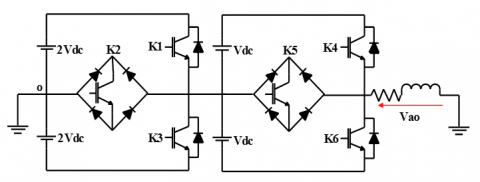Figure 1. Seven-level inverter of the proposed topology

2.1 Operation of seven-level inverter based on the proposed topology

In order to well understand how the proposed topology operates, it is imperative to define the seven-level of the output voltage Vao. The different possible level could be generated, by the association of the two three-level modules shown in Figure 1, are seven: 3Vdc, 2Vdc, Vdc, 0, -Vdc, -2Vdc and -3Vdc, as illustrated in Figure 2. For this purpose, the control process, presented in Table 1 should be followed One can be seen that each level is synthesized by a combination of the six switches where two of them are ON and others are OFF.

All possible switching combinations are analyzed in this section, as shown in Figure 3. There can be only one switching combination for each output voltage level. The current flows of these seven different cases of the proposed inverter are shown in Figure 4, where the line in red means the conduction path.

Table 1. Switching table of switches for a phase of the proposed topology

 K2 K3 K4 K5 K6 Vao IGBTs actives 1 0 0 1 0 0 3Vdc 2 1 0 0 0 1 0 2Vdc 2 0 1 0 1 0 0 Vdc 2 0 1 0 0 1 0 0 2 0 1 0 0 0 1 -Vdc 2 0 0 1 0 1 0 -2Vdc 2 0 0 1 0 0 1 -3Vdc 2

## 2.png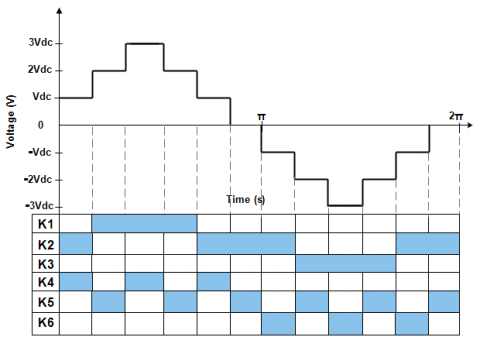Figure 2. Switching states of the proposed seven-level inverter switches

## 3a.png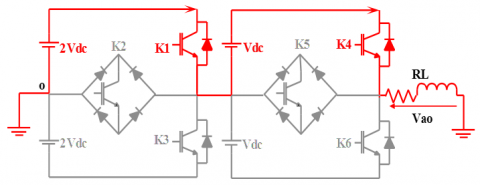(a) Vao = 3Vdc

## 3b.png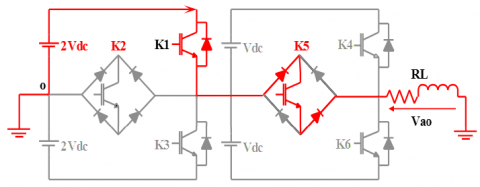(b) Vao = 2 Vdc

## 3c.png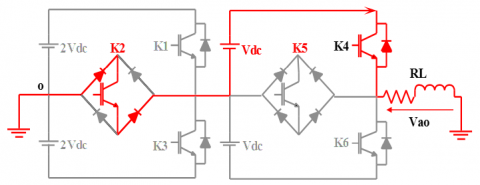(c) Vao = Vdc

## 3d.png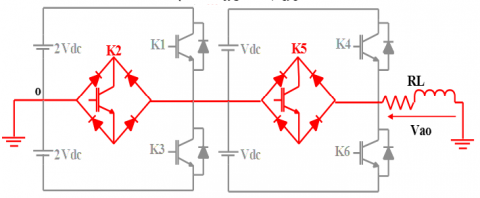(d) Vao = 0

## 3e.png(e) Vao = - Vdc

## 3f.png(f) Vao = -2 Vdc

## 3g.png(g) Vao = - 3 Vdc

Figure 3. Operating modes of single-phase seven-level inverter of the proposed topology

(1) Positive output level 3Vdc (Figure 3 (a)): In the switching case of Figure 3 (a), the total voltage applied to the load is equal to 3Vdc when the two DC sources 2Vdc and Vdc are connected in series. For the 3Vdc voltage the switches K1 and K4 must be ON and K2, K3, K5 and K6 are OFF.

(2) Positive output level 2Vdc (Figure 3 (b)): In the switching state of Figure 3 (b), the total voltage applied to the load is equal to 2Vdc. For this amplitude voltage the switches K1 and K5 are ON where and K2, K3, K4 and K6 are OFF.

(3) Positive output level 1Vdc (Figure 3 (c)): In the case of configuration of Figure 3 (b), K2 and K4 are ON where K1, K3, K5 and K6 are OFF, the total voltage applied to the load is equal to Vdc.

(4) Zero output level (Figure 3 (d)):  To get zero voltage switches K2 and K5 must be ON and K1, K3, K4 and K6 are OFF.

(5) Negative output level -1Vdc (Figure 3(e)): -Vdc is applied to the load in this switching case, switches K2 and K6 are ON where K1, K3, K4 and K5 are OFF.

(6) Negative output level -2Vdc (Figure 3(f)): In the switching state of Figure 3 (b), the total voltage applied to the load is equal to -2Vdc. for this amplitude voltage the switches K3 and K5 are ON where K1, K2, K4 and K6 are OFF.

(7) Minimum negative output level -3Vdc (Figure 3 (g)): In the switching case of Figure 3 (a), the total voltage applied to the load is equal to 3Vdc when the two DC sources 2Vdc, Vdc are connected in series. For the -3Vdc voltage K3 and K6 must be ON and K1, K2, K4 and K5 are OFF.

2.2 Extension of the proposed topology

The proposed typology can be extensible in terms of the number of levels i.e. it is possible to increase the number of levels (7, 15, 31… (2 (M + 2)-1)) by multiplying number of basic module units (Figure 4) with asymmetrical DC sources referring as illustrated in Figure 5, in this case the maximum phase voltage is given by Eq. (1):

$V_{\max }=\left(2^{(M+1)}-1\right) \cdot V_{d c}$        (1)

M is the number of modules to be added. The calculation of the number of levels N according to the number of modules to be added is given by Eq. (2):

$N=2^{(M+2)}-1$      (2)

The number of phases is also possible to increase taking into account the optimization of the DC sources number, Figure 5 illustrates the multiphase structure to this end, the structure of the proposed three-phase seven-level inverter designed from the base module (Figure 4) with an additional arm. Each arm is connected with a single base module shown in Figure 5.

## 4.png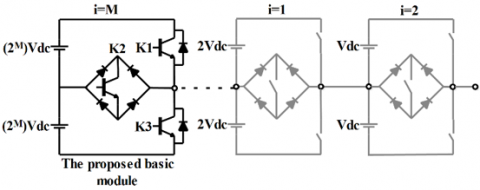Figure 4. Extension of the proposed topology in terms of level

2.3 Comparison

Many research papers, over the last decade, have dealt with seven-level inverters. Hence, the proposed topologies are compared with the relevant ones and summarized in Table 2.

Table 2. Comparison between seven-level inverters

 Topologies IGBTs Diodes Voltage sources Topology  8 12 4 Topology [8, 11] 10 10 3 Topology  12 12 3 Topology  8 10 3 Topology  8 12 3 Topology  12 12 5 Topology [14, 18] 10 10 4 Topology  8 8 4 Topology [16, 17] 16 16 3 Topology  9 9 3 Proposed topology 6 12 4

The comparison is based on the number of power components used such as the number of diodes, number of bidirectional switches and a number of DC sources.

## 5.png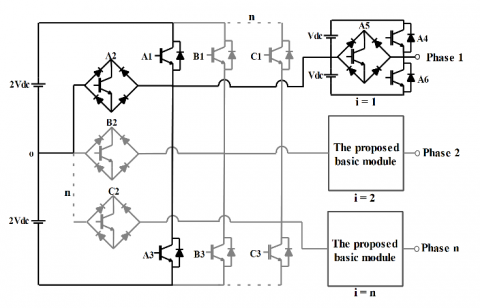Figure 5. Extension of the proposed topology in terms of phase

(1) The number of IGBT in the proposed topology is reduced compared to any topology of a seven-level, consequently reducing the cost and the complexity of the control with keeping the same waveforms quality in addition to the reduction of conducting and switching losses;

(2) The proposed topology can be generalized to N voltage levels and N voltage phases as described in section 2.2.

Moreover, the novel proposed topology of seven-level inverter keeps all advantages of the conventional multilevel inverter and eliminates their disadvantages. Compared with the NPC topologies, the proposed topology fixes the problem of neutral point balancing and the high number of capacitors. The CHB topologies have two drawbacks as the complexity of the control and the high number of isolated sources which are solved by the proposed one. Finally, the floating capacitor FC topologies contains several floating capacitors where the proposed topology is devoid of floating capacitors.

3. Simulation and Results

The proposed inverter topology and its control strategy may be tested by simulation in the following, the basic topology of the inverter can be useful is that of a single phase the results provided by seven-level single phase is presented that, in the aim of include the seven-level inverter in electrical traction drive, the three phase topology tested. Finally, performances of an electrical traction drive base of PMSM and the proposed three-phase seven-level inverter is shown.

3.1 Single-phase topology

In order to test the proposed multilevel topology, the single phase of the proposed seven-level topology feeding a load considered inductive. The control strategy of the inverter is simplified by choosing T/2 as time duration of each level where is the output voltage period (Figure 2).

The simulation parameters are as follow:

Vdc=25V and for the inductive load R= 1 Ω and L=10 mH.

Figure 6 shows the seven-level inverter with the different control signals of each IGBT over a period T and Figure 7 illustrates the voltage or terminal of the inductive load RL and the output current one can be seen that the seven voltage levels (3Vdc, 2Vdc, Vdc, 0, -Vdc, -2Vdc and -3Vdc).

## 6.png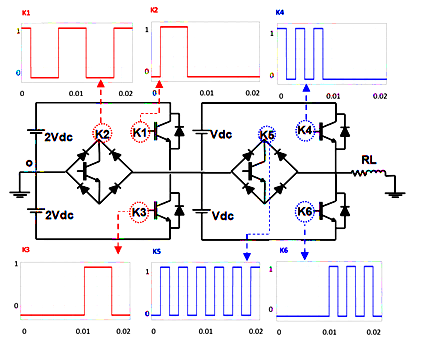Figure 6. Switching patterns of the single-phase topology

## 7.png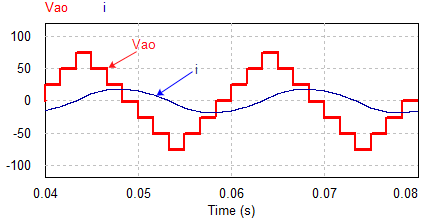Figure 7. Output phase voltage Vao and current i

## 8.png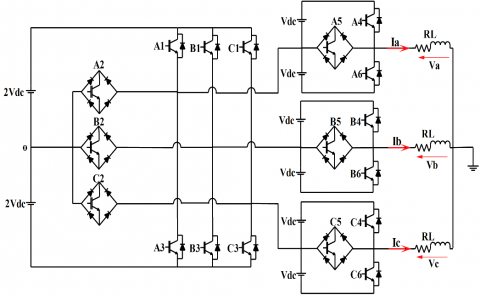Figure 8. Proposed three-phase seven-level inverter

## 9.png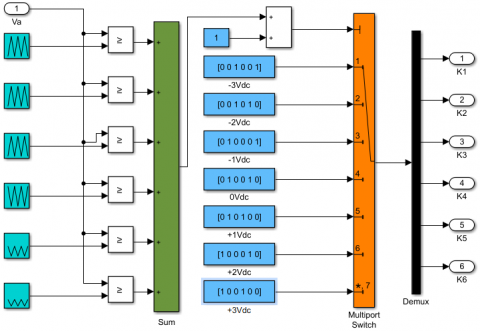Figure 9. PWM Control technique of proposed topology

3.2 Three phases seven-level inverter derived from the proposed topology

In order to show the compliance with the conditions for reducing the number of controllable power components as well as the number DC source in case of multiphase, the description of the three- phase structure is given this latter is adopted since the three-phase systems are widely used.

The structure of the proposed three-phase seven-level inverter with is presented in Figure 8. The multi-carrier PWM is the more suitable for the multilevel inverters. This is because it is chosen to control the proposed seven-level topology as depicted in Figure 9. The principle of this control is the comparison of several overlapped high frequency triangular signals and a reference sine wave of the desired output voltages. In this work, the specifically control technique used is that phase opposition disposition pulse width modulation method (PODPWM) i.e. all operators have the same frequency and amplitude, all carriers below zero are antagonistic to those above zero .

The carrier signals which are Cr1, Cr2, Cr3, Cr4, Cr5 and Cr6 with reference wave arrangements are as shown in Figure 10.

To verify the performance of the proposed seven-level three-phase inverter, simulation work was performed in the MATLAB/Simulink environment. The simulation parameters are: Vdc=400/6 V, switching frequency of 5 kHz and R= 1 Ω and L=10 mH for the inductive load.

Figures 11, 12 and 13 show the different output results of the load voltages, phase voltages, and current waveforms. Figure 12 clearly shows that the output voltage of proposed topology is a seven-level waveform. This has a direct impact on the currents waveforms which will be more interesting to show the enhancement of the performances of an electric traction drive system where it will be used.

## 10.pngFigure 10. Multi-carrier PWM

## 11.png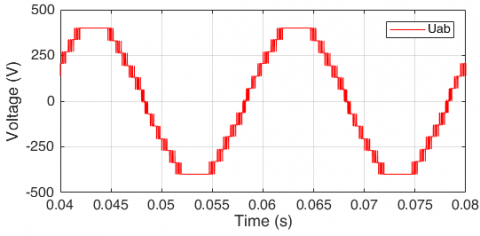Figure 11. Output line voltage Uab

## 12.png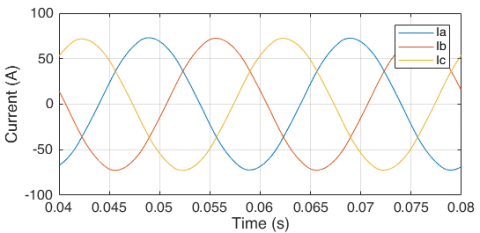Figure 12. Output phase currents Iabc

## 13.png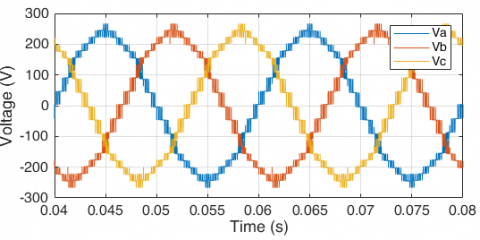Figure 13. Output phase voltage Vabc

4. Application for Electric Traction Drive Based on PMSM

The usefulness of the proposed topology can be shown by inserting it into an application. One of the promising applications is that of electric traction, case of electric vehicle

PMSMs are the most widely used in the field of motion control applications. This main is because reasons of their high-power density and high efficiency [21, 22].

The proposed seven-level inverter is used to feed the PMSM controlled by FOC technique as illustrated in Figure 14. Performances of the present global system have been verified by simulation with MATLAB Simulink.

Simulation results are shown in Figure 15-19 for 10 kHz switching frequency and PMSM parameters summarized in Table 3.

Table 3. Parameters of the permanent magnet synchronous machine

 Parameters Specification Rated Speed 3000 RPM Rated Torque 111 N.m Rated Voltage 560 V Number of Poles 4 Lq 0,000635 H Ld 0,000635 H rs 0,05 Ω Flux(φ) 0,192 Wb Inertia (J) 0,011 kg.m2 Friction 0,001889 N.m.s

## 14.png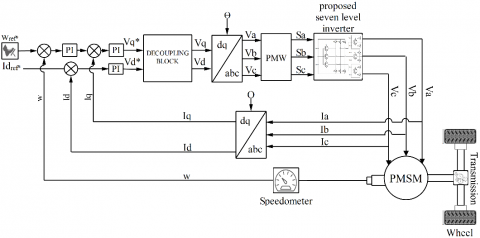Figure 14. Electric traction system based on seven-level inverter of the proposed topology

## 15a.png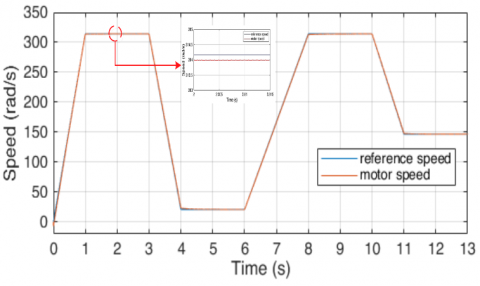(a) PMSM speed

## 15b.png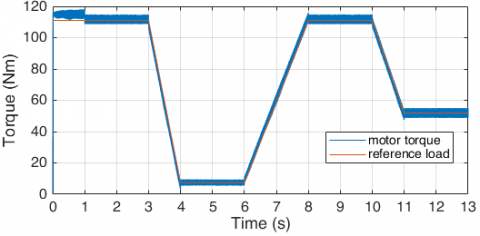(b) PMSM torque

Figure 15. PMSM speed and torque curves

## 16a.png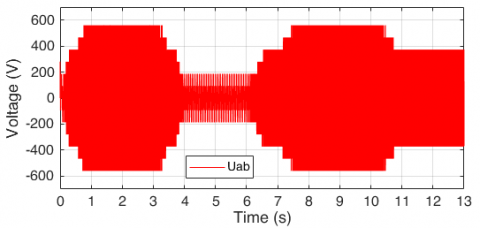(a) Line voltage Uab

## 16b.png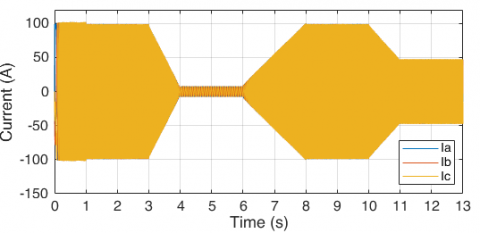(b) Phase currents Iabc

Figure 16. Line voltage Uab and phase currents Iabc waveforms

## 17a.png(a) Line voltage Uab

## 17b.png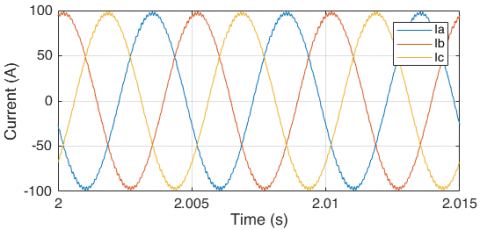(b) Phase currents Iabc

Figure 17. Line voltage Uab and phase currents Iabc waveforms at 314rad/s speed and of 111 Nm torque

## 18a.png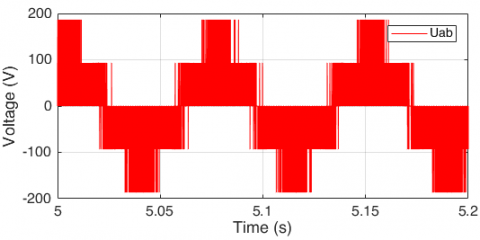(a) Line voltage Uab

## 18b.png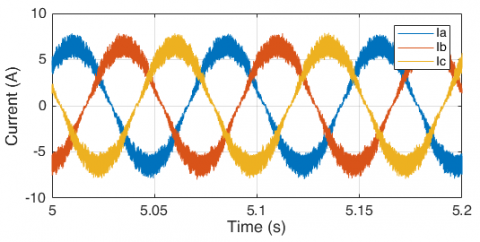(b) Phase currents Iabc

Figure 18. Line voltage Uab and phase currents Iabc waveforms at of 21 rad/s speed and of 8 Nm torque

## 19a.png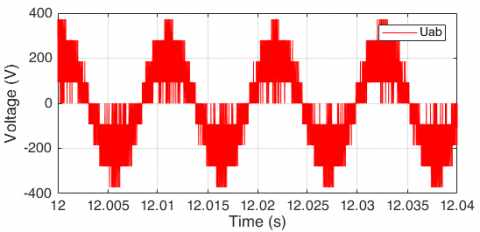(a) Line voltage Uab

## 19b.png(b) Phase currents Iabc

Figure 19. Line voltage Uab and phase currents Iabc waveforms at 147 rad/s speed and of 52 Nm torque

## 20a.png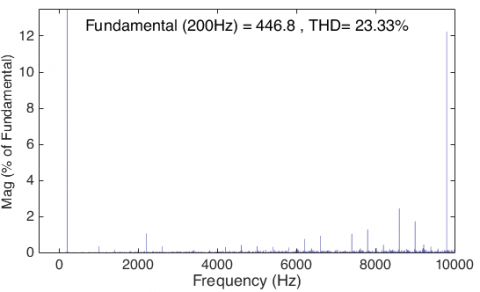## 20b.png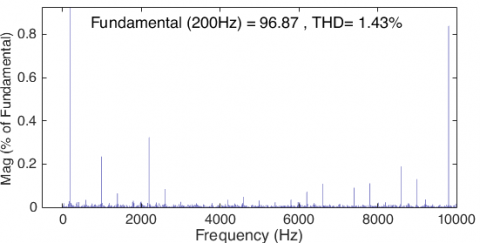Figure 20. Voltage and current harmonic distortion analysis at the synchronism speed and maximum torque

It is well known, in FOC technique, that the target control parameter is the motor speed. In our case, a speed cycle representing a different situation. Such as high, medium and low speed (Figure 14(a)) respectively (314 rad/s, 147 rad/s and 21 rad/s). Under load variation condition as depicted in Figure 14(b).

Figure 15(a) shows that the motor speed follows its reference throughout the cycle. This is because of the good waveforms of voltage provided by the seven-level inverter of the proposed topology as show in Figure 17(a), 18(a) and 19 (a). Moreover, the current (Figure 17(b), 18(b) and 19(b)) presents also sinusoidal waveforms which has a direct impact on the motor torque.

The good quality of the current waveform is resulting from that of the supplying voltage as it is illustrated in Figure 20.

5. Conclusion

This paper presents a novel multilevel inverter topology dedicated to Electric Traction drive based on proposed three-level module unit. The proposed multilevel topology provides some advantages over those already proposed in literature. The main advantages are the reduced number of controlled switches with the possible extending the number of levels and phases, and also to design a modular or compact topology. The reduced number of switches provides a costs reduction and low complexity of control. Moreover, the proposed basic module unit topology can be inserted with any other compact multilevel inverter to increase its level number.

A three phase seven-level inverter of the proposed topology is used in electric traction drive system based on PMSM with field-oriented control technique.  The obtained results, in terms of motor speed, show a good reference tracking because of the high quality of voltage and current waveforms. This will increase the battery lifetime and the reliability of the global electric traction system.

Nomenclature
 Cr Carrier DC Direct Current FC floating capacitor FOC Field Oriented Control IGBT Insulated Gate Transistors with Bipolar junction J Moment of inertia NPC Neutral Point Clamped N Number of levels M Number of modules to be added PWM Pulse Width Modulation PODPWM Phase Opposition Disposition Pulse Width Modulation THD Total Harmonic Distortion Vdc Continuous voltage
References

 Tolbert, L.M., Peng, F.Z., Habetler, T.G. (1999). Multilevel converters for large electric drives. IEEE Transactions on Industry Applications, 35(1): 36-44. https://doi.org/10.1109/28.740843

 Tolbert, L.M., Peng, F.Z., Habetler, T.G. (1998). Multilevel inverter for electric vehicle applications. Power Electronics in Transportation (Cat. No.98TH8349), Dearborn, MI, USA, pp. 79-84. https://doi.org/10.1109/PET.1998.731062

 Welchko, B.A., Nagashima, J.M. (2003). The influence of topology selection on the design of EV/HEV propulsion systems. IEEE Power Electronics Letters, 1(2): 36-40. https://doi.org/10.1109/LPEL.2003.821033

 Gautam, S.P., Kumar, L., Gupta, S. (2015). Hybrid topology of symmetrical multilevel inverter using less number of devices. IET Power Electronics, 8(11): 2125-2135. https://doi.org/10.1049/iet-pel.2015.0037

 Omer, P., Kumar, J., Surjan, B.S. (2020). A review on reduced switch count multilevel inverter topologies. IEEE Access, 8: 22281-22302. https://doi.org/10.1109/ACCESS.2020.2969551

 Rodriguez, J., Franquelo, L.G., Kouro, S., Leon, J.I., Portillo, R.C., Prats, M.A.M., Perez, M.A. (2009). Multilevel converters: An enabling technology for high-power applications. Proceedings of the IEEE, 97(11): 1786-1817. https://doi.org/10.1109/JPROC.2009.2030235

 Sun, B., Wu, F., Dragiˇcevi, T., Guerrero, J.M., Vasquez, J.C. (2014). A single-phase seven-level grid-connected inverter based on three reference SPWM strategy. 2014 IEEE International Energy Conference (ENERGYCON), Cavtat, pp. 222-227. https://doi.org/10.1109/ENERGYCON.2014.6850432

 Hinago, Y., Koizumi, H. (2012). A switched-capacitor inverter using series/parallel conversion with inductive load. IEEE Transactions on Industrial Electronics, 59(2): 878-887. https://doi.org/10.1109/TIE.2011.2158768

 Choupan, R., Golshannavaz, S., Nazarpour, D., Barmala, M. (2019). A new topology for multilevel inverters with fault-tolerant capability against open circuit faults. Electric Power Systems Research, 168: 105-116. https://doi.org/10.1016/j.epsr.2018.11.013

 Peng, W., Ni, Q., Qiu, X., Ye, Y. (2019). Seven-level Inverter with Self-Balanced Switched-Capacitor and Its Cascaded Extension. IEEE Transactions on Power Electronics, 34(12): 11889-11896. https://doi.org/10.1109/TPEL.2019.2904754

 Chen, J., Wang, C., Li, J. (2019). A single-phase step-up seven-level inverter with a simple implementation method for level-shifted modulation schemes. IEEE Access, 7: 146552-146565. https://doi.org/10.1109/ACCESS.2019.2946238

 Hsieh, C.H., Liang, T.J., Chen, S.M., Tsai, S.W. (2016). Design and implementation of a novel multilevel dc–ac inverter. IEEE Transactions on Industry Applications, 52(3): 2436-2443. https://doi.org/10.1109/TIA.2016.2527622

 Yadav, A.K., Gopakumar, K., Loganathan, U., Bhattacharya, S., Jarzyna, W. (2019). A hybrid seven-level inverter using low voltage devices and operation with single DC-link. IEEE Transactions on Power Electronics, 34(10): 9844-9853. https://doi.org/10.1109/TPEL.2018.2890371

 Sanjeevan, A.R., Kaarthik, R.S., Gopakumar, K., Rajeevan, P.P., Leon, J.I., Franquelo, L.G. (2016). Reduced common-mode voltage operation of a new seven-level hybrid multilevel inverter topology with a single DC voltage source. IET Power Electronics, 9(3): 519-528. https://doi.org/10.1049/iet-pel.2015.0130

 Siwakoti, Y., Mahajan, A., Rogers, D., Blaabjerg, F. (2019). A novel seven-level active neutral point clamped converter with reduced active switching devices and DC-link voltage. IEEE Transactions on Power Electronics, 34(11): 10492-10508. https://doi.org/10.1109/TPEL.2019.2897061

 Lee, S.S. (2018). A single-phase single-source 7-level inverter with triple voltage boosting gain. IEEE Access, 6: 30005-30011.

https://doi.org/10.1109/ACCESS.2018.2842182

 Pulikanti, S.R., Konstantinou, G., Agelidis, V.G. (2013). Hybrid seven-level cascaded active neutral-point-clamped-based multilevel converter under SHE-PWM. IEEE Transactions on Industrial Electronics, 60(11): 4794-4804. https://doi.org/10.1109/TIE.2012.2218551

 Lee, S.S., Lee, K.B. (2019). Dual-T-type seven-level boost active-neutral-point-clamped inverter. IEEE Transactions on Power Electronics, 34(7): 6031-6035. https://doi.org/10.1109/TPEL.2019.2891248

 Zeng, J., Lin, W., Liu, J. (2019). Switched-capacitor-based active-neutral-point-clamped seven-level inverter with natural balance and boost ability. IEEE Access, 7: 126889-126896. https://doi.org/10.1109/ACCESS.2019.2927351

 McGrath, B.P., Holmes, D.G. (2002). Multicarrier PWM strategies for multilevel inverters. IEEE Transactions on Industrial Electronics, 49(4): 858-867. https://doi.org/10.1109/TIE.2002.801073

 Wu, X., Zhu, Z.Q., Wu, Z., Liu, T., Li, Y. (2020). Analysis and suppression of rotor eccentricity effects on fundamental model based sensorless control of permanent magnet synchronous machine. IEEE Transactions on Industry Applications. https://doi.org/10.1109/TIA.2020.2997297

 Wang, G., Valla, M., Solsona, J. (2020). Position sensorless permanent magnet synchronous machine drives—a review. IEEE Transactions on Industrial Electronics, 67(7): 5830-5842. https://doi.org/10.1109/TIE.2019.2955409